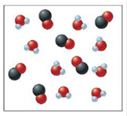Chapter 13, Problem 15Q

Chapter
Section
Textbook Problem

# Consider the following reaction at some temperature: H 2 O ( g ) + CO ( g ) ⇌ H 2 ( g ) + CO 2 ( g )             K = 2.0 Some molecules of H2O and CO are placed in a 1.0-L container as shown below.When equilibrium is reached, bow many molecules of H2O, CO, H2, and CO2 are present? Do this problem by trial and error-that is, if two molecules of CO react, is this equilibrium; if three molecules of CO react, is this equilibrium; and so on.

Interpretation Introduction

Interpretation: The reaction between water and carbon monoxide and water is given. Some of the water and carbon monoxide molecules are placed in a container. The number of molecules of H2O,CO,H2 and CO2 present at equilibrium is to be calculated. The given problem is to be solved using the trial and error method.

Concept introduction: The state when the reactants involved in a chemical reaction and the products formed in the reaction exist in concentrations having no further tendency to change is known as an equilibrium state of the reaction.

To determine: The number of molecules of H2O,CO,H2 and CO2 present at equilibrium.

Explanation

Given

The stated chemical equation is,

H2O(g)+CO(g)H2(g)+CO2(g)

Some molecules of water and carbon monoxide are placed in a 1.0L container as,

Figure 1

Case 1:

The initial molecules of H2O present =8

The initial molecules of CO present =6

On the reaction with two molecules of CO , the equilibrium reaction is,

H2O(g)+CO(g)H2(g)+CO2(g)Initialmolecules:8600Change(molecules):22+2+2Equilibrium(molecules):(82=6)(62=4)22

The equilibrium constant for the given reaction is calculated by the formula,

K=[H2][CO2][H2O][CO]

Where,

• K is the equilibrium constant.

Substitute the value of the concentration of the reactants and the products in the above expression.

K==0.17

The obtained value does not match with the given data of the reaction.

Therefore, equilibrium is not attained by the reaction of two molecules of CO with water.

Case 2:

The initial molecules of H2O present =8

The initial molecules of CO present =6

On the reaction with three molecules of CO , the equilibrium reaction is,

H2O(g)+CO(g)H2(g)+CO2(g)Initialmolecules:8600Change(molecules):33+3+3Equilibrium(molecules):(83=5)(63=3)33

The equilibrium constant for the given reaction is calculated by the formula,

K=[H2][CO2][H2O][CO]

Where,

• K is the equilibrium constant

### Still sussing out bartleby?

Check out a sample textbook solution.

See a sample solution

#### The Solution to Your Study Problems

Bartleby provides explanations to thousands of textbook problems written by our experts, many with advanced degrees!

Get Started

#### Find more solutions based on key concepts